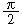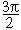home | O'Reilly's CD bookshelfs | FreeBSD | Linux | Cisco | Cisco Exam

### 2.12. Calculating More Trigonometric Functions

### Problem

You want to calculate values for trigonometric functions like sine, tangent, or arc-cosine.

### Solution

Perl provides only ``` sin``` , ``` cos``` , and ``` atan2``` as standard functions. From these, you can derive ``` tan``` and the other trig functions:

```sub tan {
my \$theta = shift;

return sin(\$theta)/cos(\$theta);
}```

The POSIX module provides a wider range of trig functions:

```use POSIX;

\$y = acos(3.7);```

The Math::Trig module provides a complete set of functions and supports operations on or resulting in complex numbers:

```use Math::Trig;

\$y = acos(3.7);```

### Discussion

The ``` tan``` function will cause a division-by-zero exception when ``` \$theta``` is,, and so on, because the cosine is 0 for these values. Similarly, ``` tan``` and many other functions from Math::Trig may generate the same error. To trap these, use ``` eval``` :

```eval {
\$y = tan(\$pi/2);
} or return undef;```

The ``` sin``` , ``` cos``` , and ``` atan2``` functions in perlfunc (1) and Chapter 3 of Programming Perl ; we talk about trigonometry in the context of imaginary numbers in Recipe 2.15 ; we talk about the use of ``` eval``` to catch exceptions in Recipe 10.122.11. Doing Trigonometry in Degrees, not Radians2.13. Taking Logarithms